# o Soil and Soil Engineering The term Soil

• Slides: 17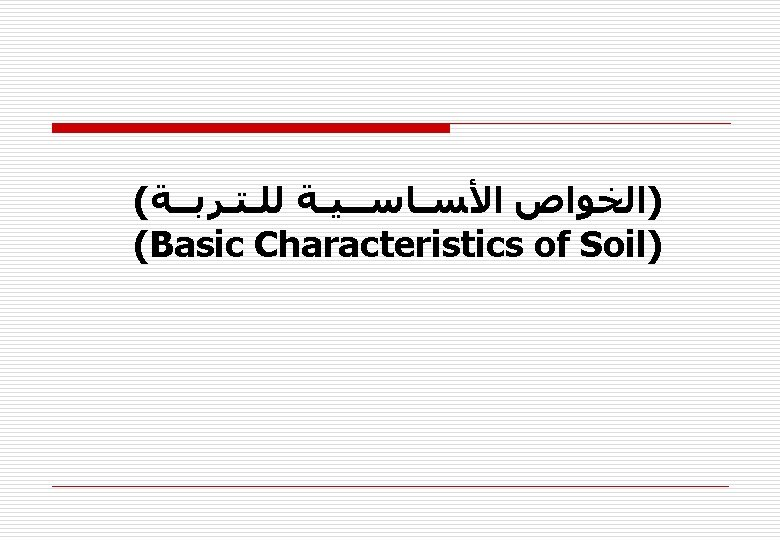o Soil and Soil Engineering *The term Soil has various meanings depending upon the general field in which it is being considered. *To a Pedologist (agricultural soil scientist). . . Soil is the substance existing on the earth's surface, which grows and develops plant life. *To a Geologist. . . Soil is the material in the thin surface zone within which roots occur, and all the rest of the earth’s crust is termed ROCK irrespective of its hardness. *To an Engineer. . Soil is the un-cemented deposits of mineral and/or organic particles or fragments covering large portion of the earth's crust.Ingredients of Soil: The nature of soil differ from other material, since it composes from three states and they respectively as follows: Solid State, Liquid State, and Gas State. The Phase Diagram In a typical volume of soil there are three components are arranged in a complex mixture: Soil Solids, Water, and Air. To visualize the relationships among the components when performing weight-volume or mass-volume calculations, drawing a phase diagram is recommended. Much like checking equilibrium with a free body diagram in statics, completing and balancing a phase diagram with numerical values of all quantities permits crosschecking the solution. The Figure below shows an element of soil and the corresponding phase diagram.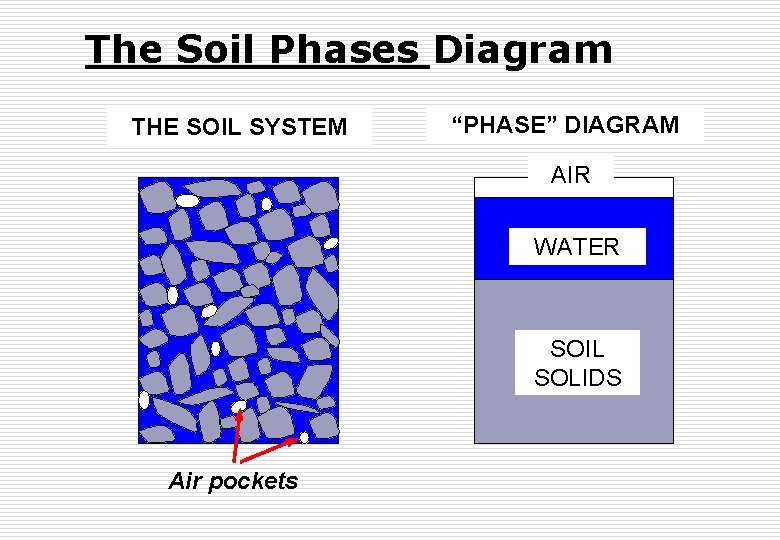The Soil Phases Diagram THE SOIL SYSTEM “PHASE” DIAGRAM AIR WATER SOIL SOLIDS Air pockets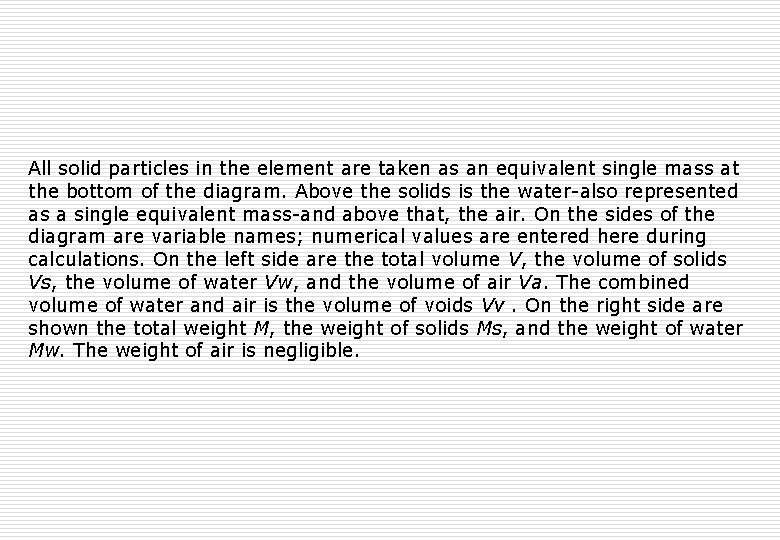All solid particles in the element are taken as an equivalent single mass at the bottom of the diagram. Above the solids is the water-also represented as a single equivalent mass-and above that, the air. On the sides of the diagram are variable names; numerical values are entered here during calculations. On the left side are the total volume V, the volume of solids Vs, the volume of water Vw, and the volume of air Va. The combined volume of water and air is the volume of voids Vv. On the right side are shown the total weight M, the weight of solids Ms, and the weight of water Mw. The weight of air is negligible.Objectives To compute the masses (or weights) and volumes of the three different phases. Notation M = mass or weight V = volume s = soil grains w = water a = air v = voids t = total Vv Va air Vw water Ma=0 Mw Vt Vs soil Phase Diagram Ms MtDefinitions Water content (w) is a measure of the water present in the soil. X 100% Expressed as percentage. Range = 0 – 100+%. Vv Va air Vw water Ma=0 Mw Vt Vs soil Phase Diagram Ms MtDefinitions Void ratio (e) is a measure of the void volume. Vv Va air Vw water Ma=0 Mw Vt Vs soil Phase Diagram Ms Mt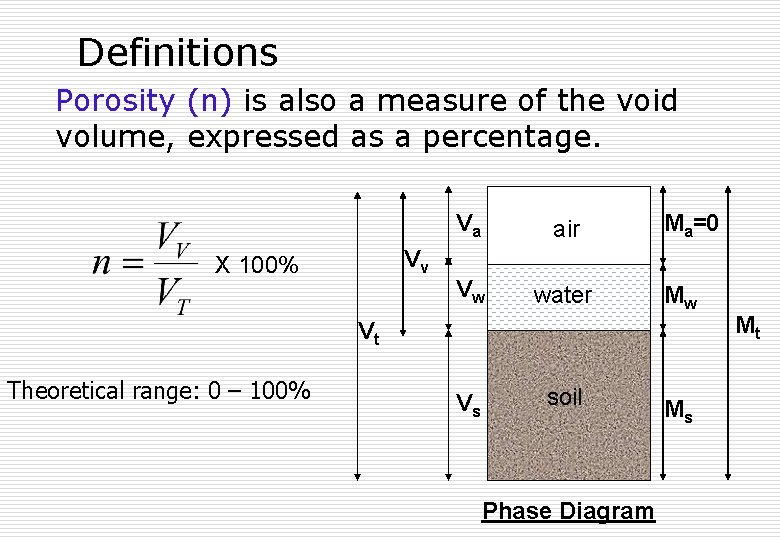Definitions Porosity (n) is also a measure of the void volume, expressed as a percentage. Vv X 100% Va air Vw water Ma=0 Mw Vt Theoretical range: 0 – 100% Vs soil Phase Diagram Ms Mt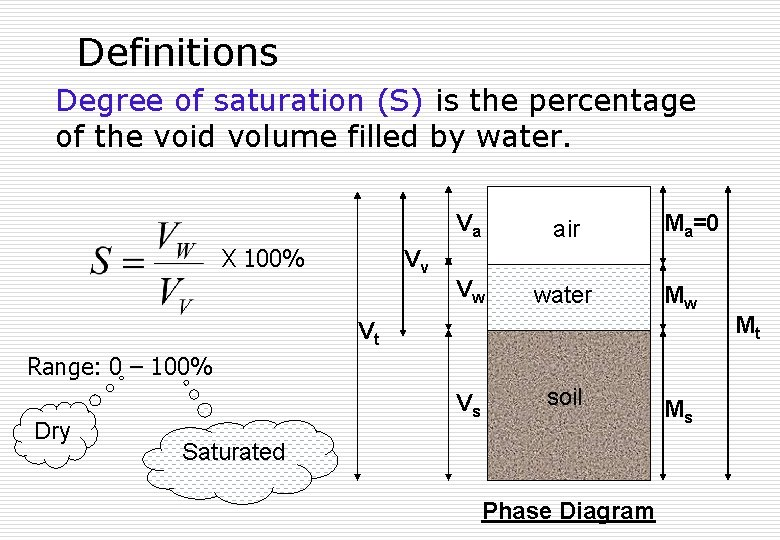Definitions Degree of saturation (S) is the percentage of the void volume filled by water. X 100% Vv Va air Vw water Ma=0 Mw Vt Range: 0 – 100% Dry Vs soil Saturated Phase Diagram Ms Mt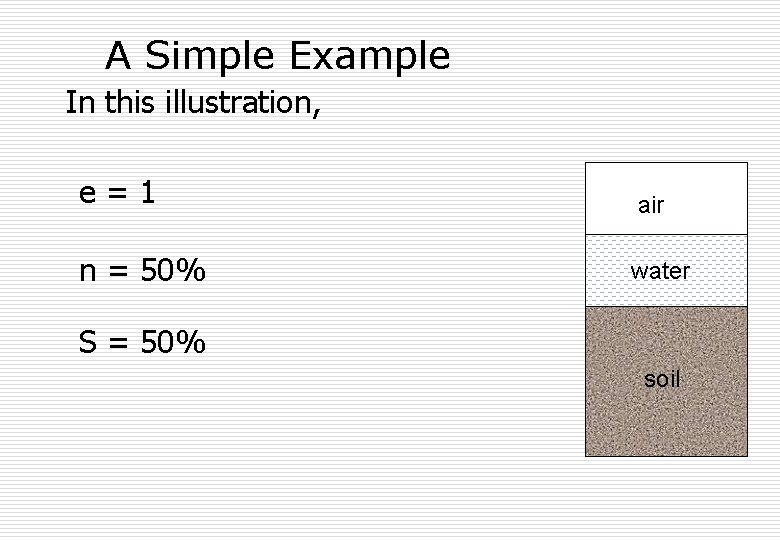A Simple Example In this illustration, e=1 air n = 50% water S = 50% soil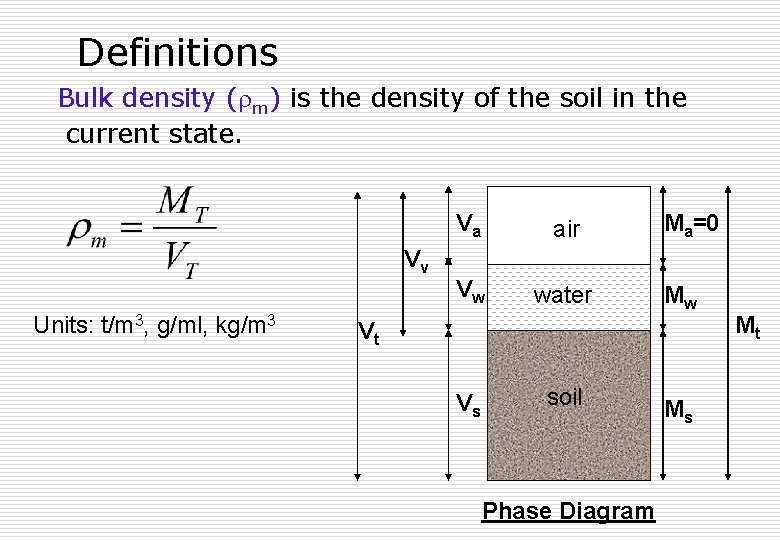Definitions Bulk density ( m) is the density of the soil in the current state. Vv Units: t/m 3, g/ml, kg/m 3 Va air Vw water Ma=0 Mw Vt Vs soil Phase Diagram Ms Mt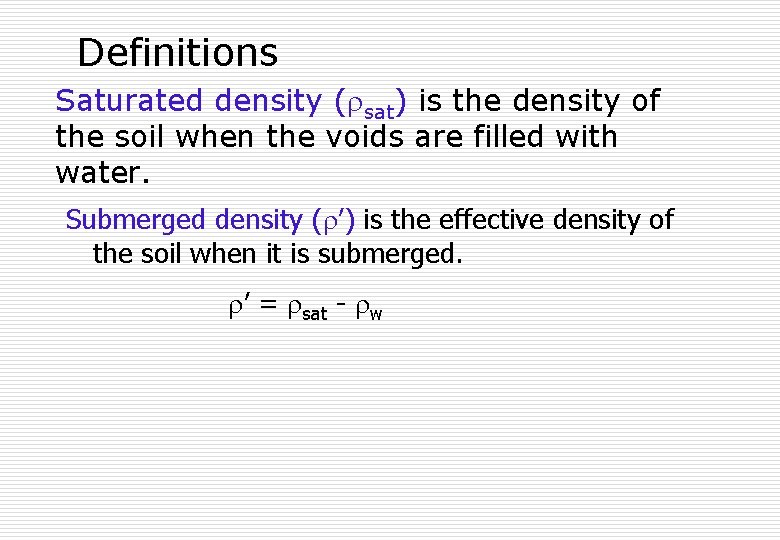Definitions Saturated density ( sat) is the density of the soil when the voids are filled with water. Submerged density ( ’) is the effective density of the soil when it is submerged. ’ = sat - w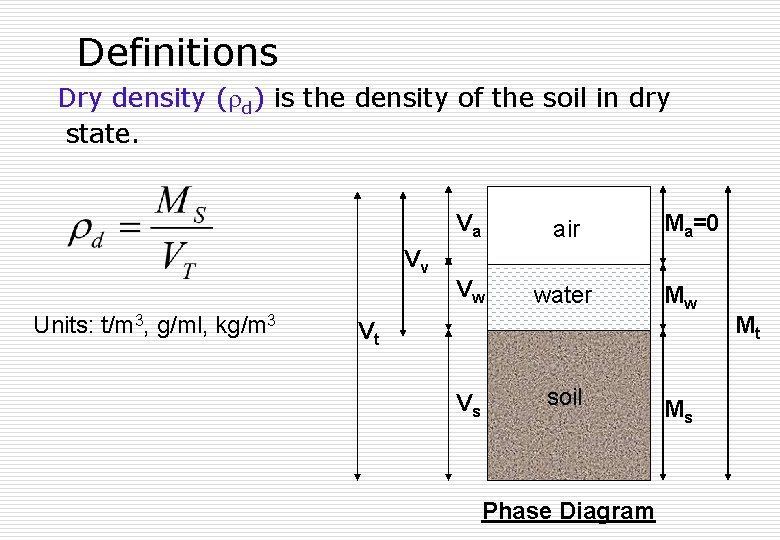Definitions Dry density ( d) is the density of the soil in dry state. Vv Units: t/m 3, g/ml, kg/m 3 Va air Vw water Ma=0 Mw Vt Vs soil Phase Diagram Ms MtDefinitions Bulk, saturated, dry and submerged unit weights ( ) are defined in a similar manner. = g N/m 3 kg/m 3 m/s 2 Specific gravity of the soil grains (Gs) typically varies between 2. 6 and 2. 8.Testing Times… State whether the following are true or false? (a) Dry density is greater than submerged density (b) In unsaturated soils, water content is always less than 100%. True (c) Larger the void ratio, larger the porosity. True False